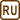## The Mixing Problem

### Task number: 1795

There are 3 kg of water at a temperature of 10 °C in a vessel. How much water at a temperature of 90 °C should we add so that the resultant temperature of water in the vessel is 35 °C? Heat capacity of the vessel is not considered.

• #### Hint

Think over what will happen with the heat after pouring the warm water and how the resultant water temperature depends on the amount of added water.

• #### Analysis

By pouring warmer water into the colder one we increase the resultant water temperature in the vessel because the warmer water supplies heat to the colder water. As long as we neglect the heat capacity of the vessel, the heat supplied by warmer water is equal to the heat received by cooler water. From the formed equality the required mass of warm water can be expressed.

• #### Notation

 m1 = 3 kg mass of colder water t1 = 10 °C temperature of colder water t2 = 90 °C temperature of warmer water tr = 35 °C resultant water temperature m2 = ? mass of added water
• #### Solution

From the equality of supplied and received heat we get the following equation

$Q_{supplied}=Q_{received},$ $m_{2}c_{w}\left( t_{2}-t_{r}\right) = m_{1}c_{w}\left( t_{r}-t_{1}\right),$

where m1 is the mass of water in the vessel, t1 is its temperature, t2 is the temperature of added water, tv is the steady state temperature and m2 is the unknown mass of added water, which we can express as follows

$m_{2}=\frac{m_{1}\left( t_{r}-t_{1}\right)}{t_{2}-t_{r}}.$
• #### Numeric substitution

$m_{2}=\frac{m_{1}\left( t_{r}-t_{1}\right)}{t_{2}-t_{r}}=\frac{3\cdot \left( 35-10\right)}{90-35}\, \mathrm{kg}$ $m_{2}=1.4\, \mathrm{kg}$
• #### Answer

To obtain the desired temperature we have to add approximately 1.4 kg of water.

• #### Comment

As we see in the solution, we do not need to know the specific heat capacity of water. The problem can be computed by the Rule of Three. We may realize that the more warm water we pour, to the lesser extent it cools. It means that the mass of water and the difference of the temperatures before mixing and after that are inversely proportional. Therefore, we can write the following equation

$\frac{m_{2}}{m_{1}} = \frac{t_{r} - t_{1}}{t_{2} - t_{r}},$

from which we can easily express the required mass m2.